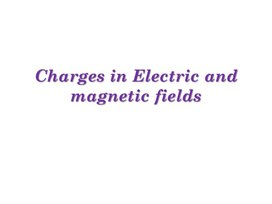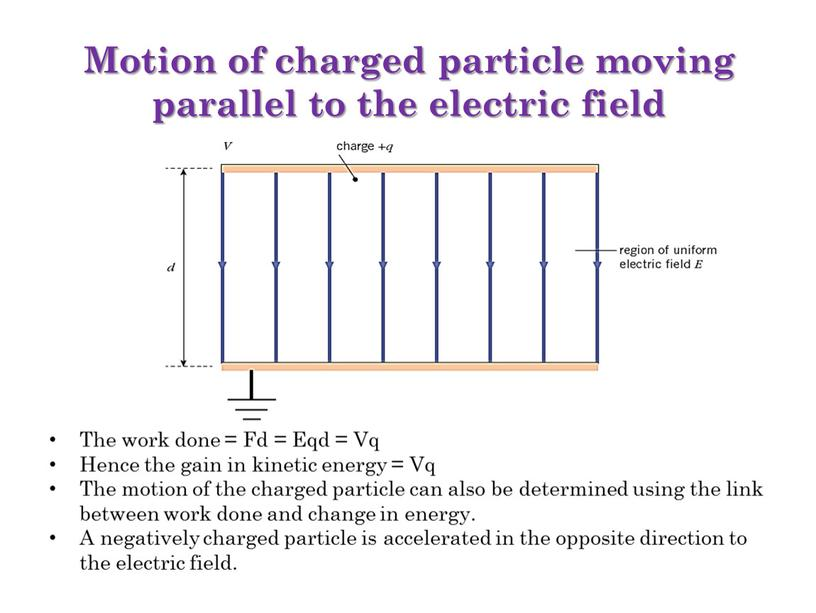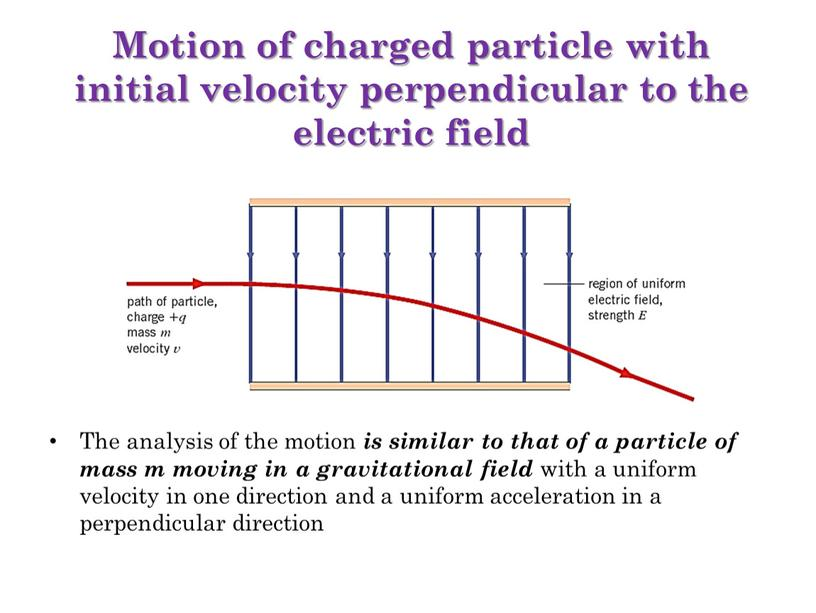Charges in Electric and magnetic fields
Оценка 5

# Charges in Electric and magnetic fields

Оценка 5
pptx
07.05.2020Charges in Electric and magnetic fields.pptx

Charges in Electric and magnetic fields

## Motion of charged particle moving parallel to the electric fieldMotion of charged particle moving parallel to the electric field

The work done = Fd = Eqd = Vq
Hence the gain in kinetic energy = Vq
The motion of the charged particle can also be determined using the link between work done and change in energy.
A negatively charged particle is accelerated in the opposite direction to the electric field.

## Motion of charged particle with initial velocity perpendicular to the electric fieldMotion of charged particle with initial velocity perpendicular to the electric field

The analysis of the motion is similar to that of a particle of mass m moving in a gravitational field with a uniform velocity in one direction and a uniform acceleration in a perpendicular direction

1.

2.

3.

4.

Скачать файл